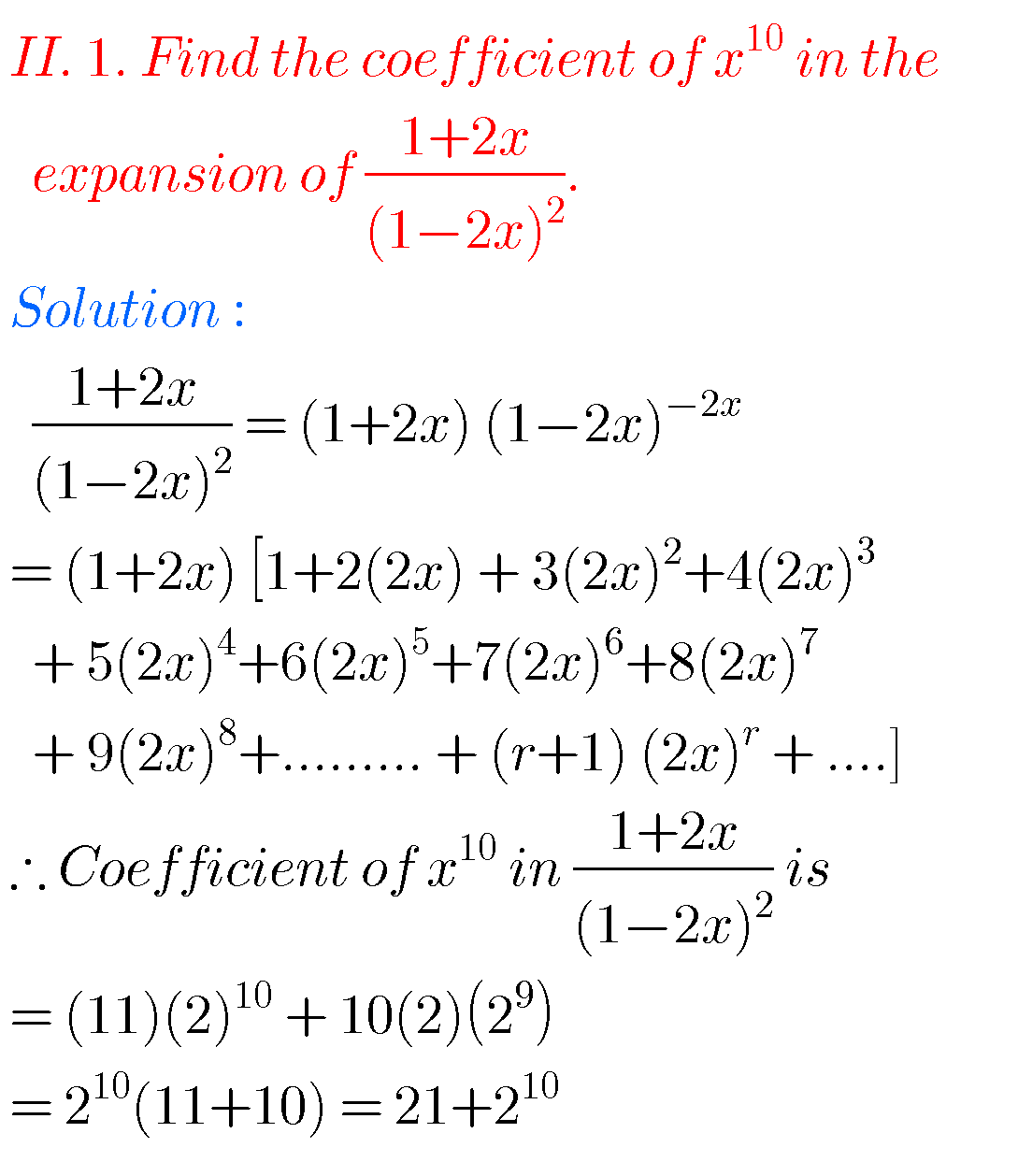## Binomial Theorem Solutions Inter second year Maths

Binomial Theorem solutions Inter second year Maths Intermediate mathematics IIA chapter 6 Binomial Theorem exercises 6(a), 6(b) and 6(c) solutions are given. These solutions are very easy to understand.  First study the text book lesson Binomial Theorem very well.  Then observe solutions and try them in your own method. You can also see Inter Maths …